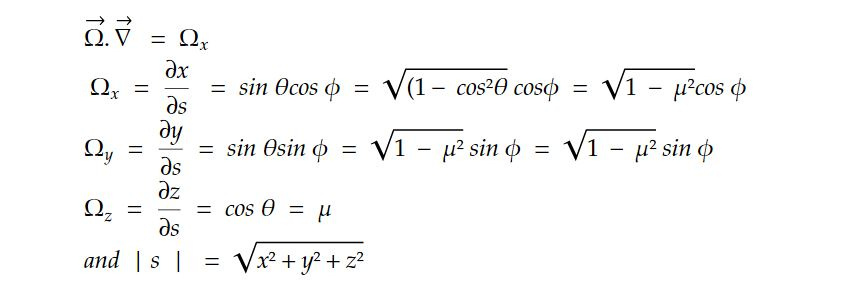# Radiative Transfer equation for 3 dimension in homogeneous medium

The radiative transfer equation is a fundamental equation in physics and astrophysics that describes how electromagnetic radiation (such as light) propagates through a medium and interacts with that medium. It is used to model the transport of radiation in various contexts, including astrophysics, atmospheric science, remote sensing, and engineering.

For most of atmosphere, phase parallel atmosphere assumption is not valid. In such a case, spherical geometry of atmosphere is applied. The general radiative transfer equation isLet extinction coefficient 𝛽_e = 𝜌K_𝜆 and omitting subscript 𝜆 for simplicity. We have

- dI/ 𝛽edS = I - J

The operator d/dS in terms of time and space is given bywhere c is velocity of light

Ω is a unit vector specifying the direction of scattering through position vector dS

In steady state, radiation is independent of time such as illumination from the sum and ∂/ ∂t = 0Now, RTE can be expressed aswhere J gives scattering of direct solar beam, multiple scattering of diffused intensity and emission by the medium.

In cartesian coordinates (x, y, z) we haveThis note is a part of the Physics Repository.